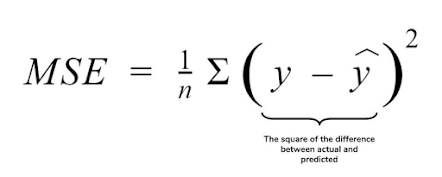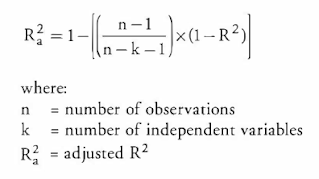Raghav Agrawal — Published On May 19, 2021 and Last Modified On July 21st, 2022

This article was published as a part of the Data Science Blogathon

## Introduction

Machine Learning is a branch of Artificial Intelligence. It contains many algorithms to solve various real-world problems. Building a Machine learning model is not only the Goal of any data scientist but deploying a more generalized model is a target of every Machine learning engineer.

Regression is also one type of supervised Machine learning and in this tutorial, we will discuss various metrics for evaluating regression Models and How to implement them using the sci-kit-learn library.

• Regression
• Why we require Evaluation Metrics
• Mean Absolute Error(MAE)
• Mean Squared Error(MSE)
• RMSE
• RMSLE
• R squared
• EndNote

## Regression

Regression is a type of Machine learning which helps in finding the relationship between independent and dependent variable.

In simple words, Regression can be defined as a Machine learning problem where we have to predict discrete values like price, Rating, Fees, etc.

## Why We require Evaluation Metrics?

Most beginners and practitioners most of the time do not bother about the model performance. The talk is about building a well-generalized model, Machine learning model cannot have 100 per cent efficiency otherwise the model is known as a biased model. which further includes the concept of overfitting and underfitting.

It is necessary to obtain the accuracy on training data, But it is also important to get a genuine and approximate result on unseen data otherwise Model is of no use.

So to build and deploy a generalized model we require to Evaluate the model on different metrics which helps us to better optimize the performance, fine-tune it, and obtain a better result.

If one metric is perfect, there is no need for multiple metrics. To understand the benefits and disadvantages of Evaluation metrics because different evaluation metric fits on a different set of a dataset.

Now, I hope you get the importance of Evaluation metrics. let’s start understanding various evaluation metrics used for regression tasks.

### Dataset

For demonstrating each evaluation metric using the sci-kit-learn library we will use the placement dataset which is a simple linear dataset that looks something like this.

Now I am applying linear regression on the particular dataset and after that, we will study each evaluation metric and check it on our Linear Regression model.

let’s start Exploring various Evaluation metrics.

## 1) Mean Absolute Error(MAE)

MAE is a very simple metric which calculates the absolute difference between actual and predicted values.

To better understand, let’s take an example you have input data and output data and use Linear Regression, which draws a best-fit line.

Now you have to find the MAE of your model which is basically a mistake made by the model known as an error. Now find the difference between the actual value and predicted value that is an absolute error but we have to find the mean absolute of the complete dataset.

so, sum all the errors and divide them by a total number of observations And this is MAE. And we aim to get a minimum MAE because this is a loss.

• The MAE you get is in the same unit as the output variable.
• It is most Robust to outliers.

• The graph of MAE is not differentiable so we have to apply various optimizers like Gradient descent which can be differentiable.
```from sklearn.metrics import mean_absolute_error
print("MAE",mean_absolute_error(y_test,y_pred))```

Now to overcome the disadvantage of MAE next metric came as MSE.

## 2) Mean Squared Error(MSE)

MSE is a most used and very simple metric with a little bit of change in mean absolute error. Mean squared error states that finding the squared difference between actual and predicted value.

So, above we are finding the absolute difference and here we are finding the squared difference.

What actually the MSE represents? It represents the squared distance between actual and predicted values. we perform squared to avoid the cancellation of negative terms and it is the benefit of MSE.The graph of MSE is differentiable, so you can easily use it as a loss function.

• The value you get after calculating MSE is a squared unit of output. for example, the output variable is in meter(m) then after calculating MSE the output we get is in meter squared.
• If you have outliers in the dataset then it penalizes the outliers most and the calculated MSE is bigger. So, in short, It is not Robust to outliers which were an advantage in MAE.
```from sklearn.metrics import mean_squared_error
print("MSE",mean_squared_error(y_test,y_pred))```

## 3) Root Mean Squared Error(RMSE)

As RMSE is clear by the name itself, that it is a simple square root of mean squared error.

•  The output value you get is in the same unit as the required output variable which makes interpretation of loss easy.

• It is not that robust to outliers as compared to MAE.

for performing RMSE we have to NumPy NumPy square root function over MSE.

`print("RMSE",np.sqrt(mean_squared_error(y_test,y_pred)))`

Most of the time people use RMSE as an evaluation metric and mostly when you are working with deep learning techniques the most preferred metric is RMSE.

## 4) Root Mean Squared Log Error(RMSLE)

Taking the log of the RMSE metric slows down the scale of error. The metric is very helpful when you are developing a model without calling the inputs. In that case, the output will vary on a large scale.

To control this situation of RMSE we take the log of calculated RMSE error and resultant we get as RMSLE.

To perform RMSLE we have to use the NumPy log function over RMSE.

`print("RMSE",np.log(np.sqrt(mean_squared_error(y_test,y_pred))))`

It is a very simple metric that is used by most of the datasets hosted for Machine Learning competitions.

## 5) R Squared (R2)

R2 score is a metric that tells the performance of your model, not the loss in an absolute sense that how many wells did your model perform.

In contrast, MAE and MSE depend on the context as we have seen whereas the R2 score is independent of context.

So, with help of R squared we have a baseline model to compare a model which none of the other metrics provides. The same we have in classification problems which we call a threshold which is fixed at 0.5. So basically R2 squared calculates how must regression line is better than a mean line.

Hence, R2 squared is also known as Coefficient of Determination or sometimes also known as Goodness of fit.

Now, how will you interpret the R2 score? suppose If the R2 score is zero then the above regression line by mean line is equal means 1 so 1-1 is zero. So, in this case, both lines are overlapping means model performance is worst, It is not capable to take advantage of the output column.

Now the second case is when the R2 score is 1, it means when the division term is zero and it will happen when the regression line does not make any mistake, it is perfect. In the real world, it is not possible.

So we can conclude that as our regression line moves towards perfection, R2 score move towards one. And the model performance improves.

The normal case is when the R2 score is between zero and one like 0.8 which means your model is capable to explain 80 per cent of the variance of data.

```from sklearn.metrics import r2_score
r2 = r2_score(y_test,y_pred)
print(r2)```

The disadvantage of the R2 score is while adding new features in data the R2 score starts increasing or remains constant but it never decreases because It assumes that while adding more data variance of data increases.

But the problem is when we add an irrelevant feature in the dataset then at that time R2 sometimes starts increasing which is incorrect.

Hence, To control this situation Adjusted R Squared came into existence.Now as K increases by adding some features so the denominator will decrease, n-1 will remain constant. R2 score will remain constant or will increase slightly so the complete answer will increase and when we subtract this from one then the resultant score will decrease. so this is the case when we add an irrelevant feature in the dataset.

And if we add a relevant feature then the R2 score will increase and 1-R2 will decrease heavily and the denominator will also decrease so the complete term decreases, and on subtracting from one the score increases.

```n=40
k=2

Hence, this metric becomes one of the most important metrics to use during the evaluation of the model.

## EndNote

I hope it was easy to catch all the important 6 metrics we have discussed. There is not anyone metric that always performs well and helps to build the generalized model.

There can be situations where you have to use different evaluation metrics and if you are a beginner then you should try all these metrics which will help you to get a better understanding of each to evaluate when you can use which metric.

I would encourage you to pick any dataset, apply a Machine learning algorithm and try to evaluate a model on different evaluation metrics.

The media shown in this article are not owned by Analytics Vidhya and is used at the Author’s discretion.